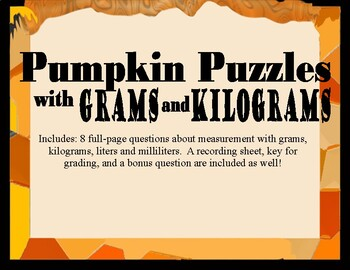# Pumpkin Puzzles SCOOT with Grams, Kilograms, Liters, and Milliliters ActivitySubject
Resource Type
File Type

PDF

(1019 KB)
Product Rating
Standards
• Product Description
• StandardsNEW

Metric Measurement is kids is always a bit challenging, and I created this to incorporate some fall fun into our thinking!

The SCOOT includes 8 full-page word problems and one bonus question. The problems use addition, subtraction, multiplication, and division, and even feature two-step thinking to solve. Included you'll find a recording sheet and key for your use as well.

Use multiplication and division within 100 to solve word problems in situations involving equal groups, arrays, and measurement quantities, e.g., by using drawings and equations with a symbol for the unknown number to represent the problem.
Interpret whole-number quotients of whole numbers, e.g., interpret 56 ÷ 8 as the number of objects in each share when 56 objects are partitioned equally into 8 shares, or as a number of shares when 56 objects are partitioned into equal shares of 8 objects each. For example, describe a context in which a number of shares or a number of groups can be expressed as 56 ÷ 8.
Interpret products of whole numbers, e.g., interpret 5 × 7 as the total number of objects in 5 groups of 7 objects each. For example, describe a context in which a total number of objects can be expressed as 5 × 7.
Measure and estimate liquid volumes and masses of objects using standard units of grams (g), kilograms (kg), and liters (l). Add, subtract, multiply, or divide to solve one-step word problems involving masses or volumes that are given in the same units, e.g., by using drawings (such as a beaker with a measurement scale) to represent the problem.
Total Pages
N/A
N/A
Teaching Duration
N/A
Report this Resource to TpT
Reported resources will be reviewed by our team. Report this resource to let us know if this resource violates TpT’s content guidelines.